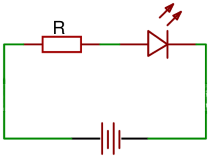# LED resistor calculator

Calculator and formulas for calculating the LED series resistor

### Calculate the LED series resistor

LEDs are usually operated via a series resistor. The series resistor must be dimensioned in such a way that the supply voltage and the maximum permissible current are not exceeded. You can calculate the size of the resistor on this page.

LED resistor calculator

 Input Source voltage V LED voltage V LED current µA mA A Decimal places 0 1 2 3 4 6 8 10 Results Series resistor Rv Resistor Power Pv### Legende

$$\displaystyle V_S$$ Source voltage

$$\displaystyle V_D$$  LED voltage

$$\displaystyle I_D$$ Maximum LED current

$$\displaystyle R_V$$ Serial resistance

$$\displaystyle P_V$$ Serial resistance power rating

## Formulas for LED series resistor

 Serial resistance $$\displaystyle R_v=\frac{V_S - V_D}{I_D}$$ Serial resistance power rating $$\displaystyle P_v=(V_S - V_D) · I_D$$ $$\displaystyle =I_D^2 · R_v$$